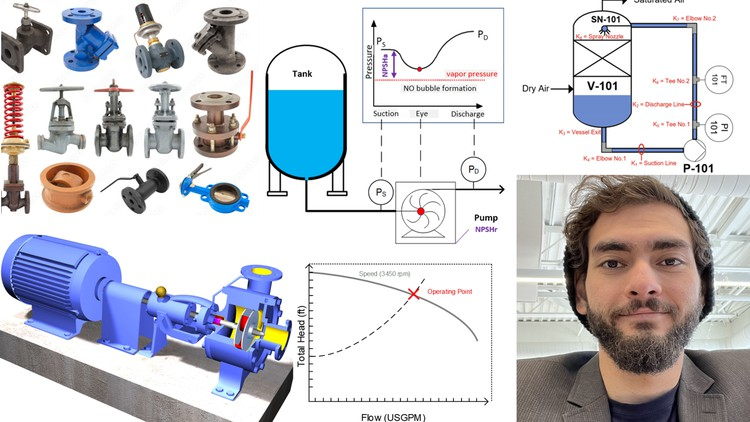# Pump Sizing & Modeling Piping Systems For LiquidsLearn to size pumps and model piping systems & valves, calculate pressure drop through fittings, valves and components

What you will learn

### Understand inherent valve curves, different types of control valves, valve authority, and size valves appropriately for a given system.

Description

Chapter 1: Introduction

1. Differentiate between incompressible and compressible fluids using the continuity equation.

2. Define and understand general concepts in fluid dynamics, such as viscosity (dynamic and kinematic), Reynolds number (laminar and turbulent flow), and volumetric flow rate.

Chapter 2: Energy Balance

3. Derive and apply the Bernoulli equation to develop an energy balance for sizing pumps.

4. Understand the pump equation and its relation to the Bernoulli equation.

5. Apply the pump equation to an illustrative example.

6. Create system curves for a piping system.

7. Understand hydraulic resistances in pipes.

8. Define the Darcy equation and its application in calculating frictional headloss.

9. Define the resistance coefficient (K) and calculate it using different methods.

10. Define the friction factor (f) and calculate it using numerical methods via the Poiseuille equation, Colebrook equation, Swamee Jain equation, or the Moody chart.

11. Understand the effect of pipe age on friction factor.

12. Define flow coefficient (Cv) and its application in calculating pressure drop.

13. Adjust Cv for liquids with different viscosities.

14. Find the capacity flow rate at different pressure drops for a given Cv.

15. Convert flow coefficient (Cv) to a resistant coefficient (K).

16. Understand the use of orifice plates and use the orifice design equation to size orifice plates.

17. Apply the orifice design equation in an illustrative example.

Chapter 4: Pumps

18. Identify and understand the basic components of a pump.

19. Calculate pumps Hydraulic Horsepower (HHP), Brake Horsepower (BHP), Pump Efficiency, Motor Power (MP), and Motor Efficiency through an example.

20. Understand pump curves (head vs flow rate) for different impeller speeds (or diameters).

21. Understand pump efficiency curves.

22. Understand Pumps Net Positive Suction Head Required (NPSHr) Curve.

23. Understand Pump Power Consumption Curve.

Chapter 5: System Modeling and Pump Sizing Roadmap

24. Model resistance in series for a piping system.

25. Develop a roadmap for proper selection of pumps.

Chapter 6: Case Study 1

26. Apply the pump sizing roadmap using Macros in Excel to select an appropriate pump for a real-world case study.

Chapter 7: Control Valves

27. Identify different types of control valves and their applications.

28. Understand the inherent valve curve and its relation to flow rate and pressure drop.

29. Define valve authority and its significance in valve selection.

30. Size valves appropriately for a given system.

English
language

Content

### Introduction

Teaching Style
Incompressible vs Compressible Fluids
General Definitions: Viscosity (Dynamic vs Kinematic)
General Definition: Reynolds Number (Laminar vs Turbulent Flow)
General Definition: Flowrate

### Energy Balance

Bernoulli’s Principle: Fluid Total Energy
Bernoulli’s Principle: Energy Terms &Units
Pipe Energy Balance Derivation Part 1
Pipe Energy Balance Derivation Part 2
Pipe Energy Balance Units
Pump Energy Balance
Example (Problem Statement)
Example (Solution Part 1)
Example (Solution Part 2)
Example (Solution Part 3) – System Curves

Darcy Equation, Resistance Coefficient
Resistance Coefficient Examples
Friction Factor
Moody Chart
Effect of Pipe Age on Friction Factor
Flow Coefficient Cv
Flow Coefficient Cv – viscosity and specific gravity correction
Flow Coefficient Cv – capacity flowrate at different pressure drops
Flow Coefficient Cv – Conversion to Resistance Coefficient K
Orirfice Plate
Orifice Plate Example

### Pumps

Pump Intro
Pump Parts
Pump Power Losses
Pump HHP, BHP,MP Efficiency
Pump HHP, BHP,MP Efficiency Example
Pump Curve
Pump Performance Curves
Pump Performance Curves Example
Pump VFDs
NPSH
Affinity Laws

### System Modeling and Pump Sizing Roadmap

Resistances in Series

### Case Study I

Case Study 1 – Problem Statement
Case Study 1 – Solution Road Map
Case Study 1 – Solution (Part 1)
Case Study 1 – Solution (Part 2)
Case Study 1 – Solution (Part 3)
Case Study 1 – Solution (Part 4)
Case Study 1 – Solution (Part 5)
Case Study 1 – Solution (Part 6)
Case Study 1 – Solution (Part 7)
Case Study 1 – Solution (Part 8)

### Control Valves

Valve Basic Parts
Valve Classification
Control Valves
Inherit Valve Curve
Inherit Valve Curve Example
Valve Authority